# Scatter plot with marginal histograms in seaborn

## Marginal histograms with jointplot

With the jointplot function is straightforward to create a scatter plot (and other types of plots) with marginal histograms. You will need to input your variables to create the visualization.

import seaborn as sns

sns.jointplot(data = df,
x = "bill_length_mm",
y = "bill_depth_mm")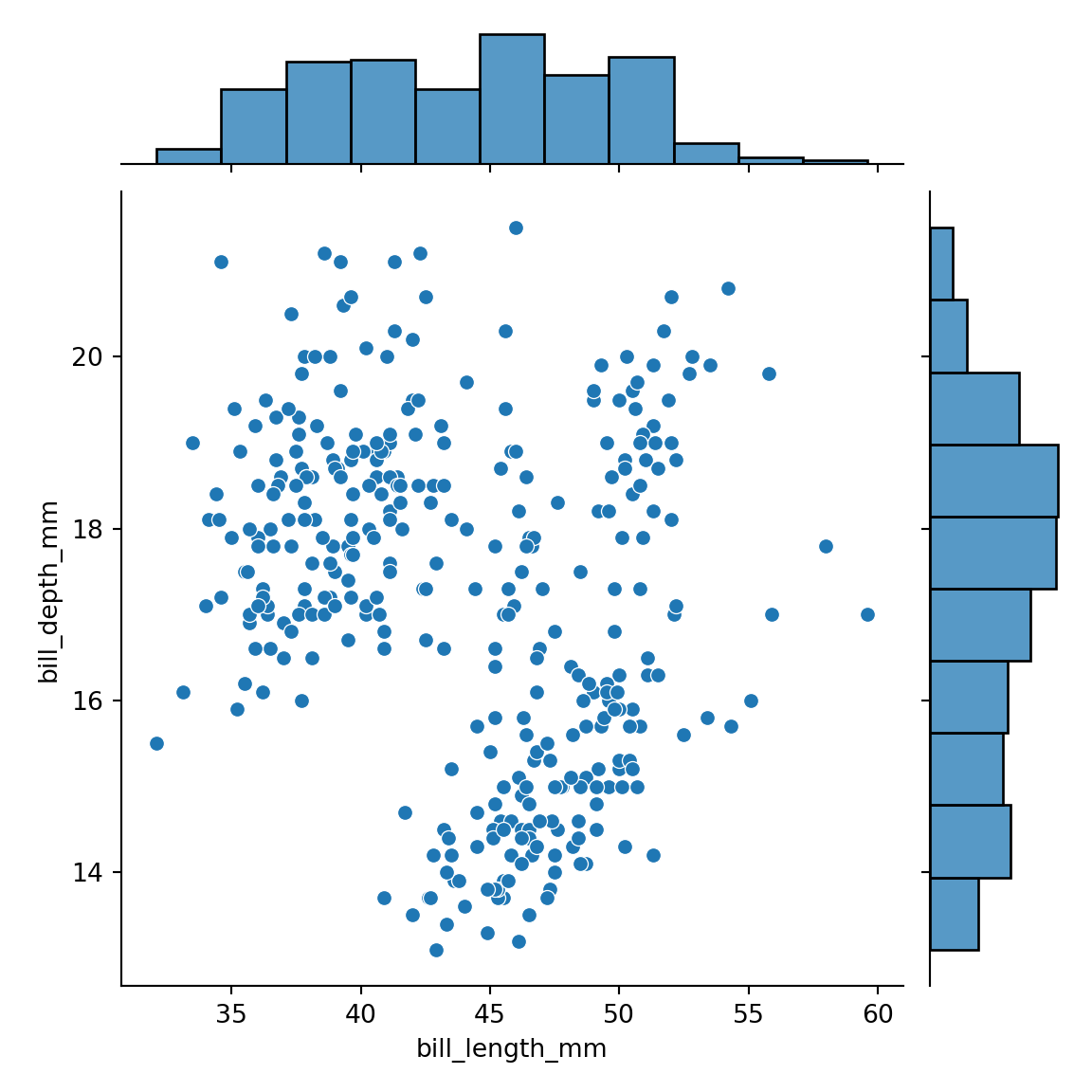An alternative to the jointplot function is JointGrid, which will provide more flexibility, if needed. jointgrid is just a lightweight wrapper of that function.

import seaborn as sns

ax = sns.JointGrid(data = df,
x = "bill_length_mm",
y = "bill_depth_mm")

ax.plot(sns.scatterplot, sns.histplot)Adding a regression line and densities

The function provides an argument named kind that allows you to select between different kind of plots. Possible options are "scatter" (default), "kde", "hist", "hex", "reg" and "resid".

import seaborn as sns

sns.jointplot(data = df,
x = "bill_length_mm",
y = "bill_depth_mm",
kind = "reg") # Adds regression and densities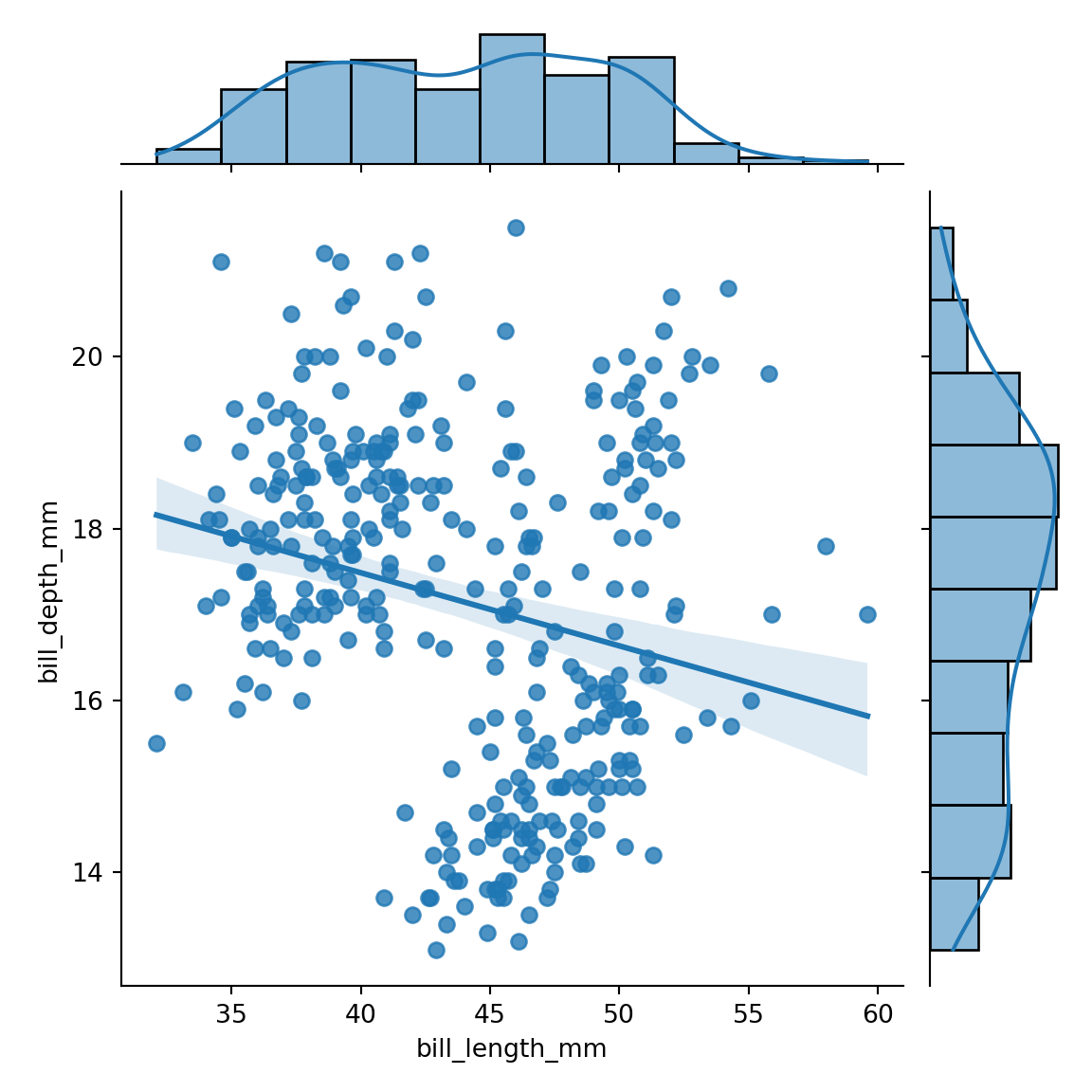Custom marker

The marker (symbol) used to represent the observations of the scatter plot can be customized with the marker argument.

import seaborn as sns

sns.jointplot(data = df,
x = "bill_length_mm",
y = "bill_depth_mm",
marker = "x")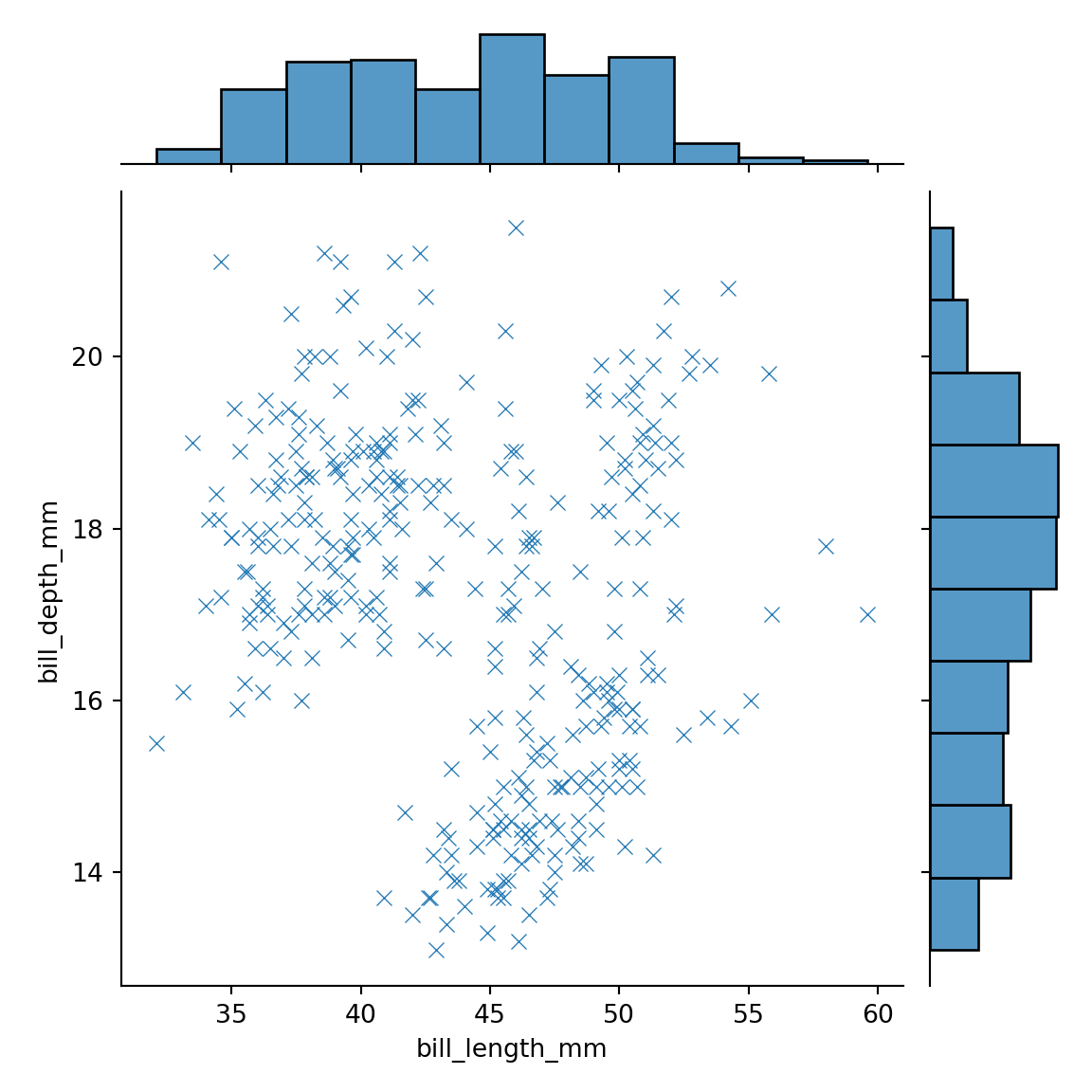Display the axes of the marginal plots

If you want the axes of the marginal plots to be displayed you can set the marginal_ticks argument to True.

import seaborn as sns

sns.jointplot(data = df,
x = "bill_length_mm",
y = "bill_depth_mm",
marginal_ticks = True)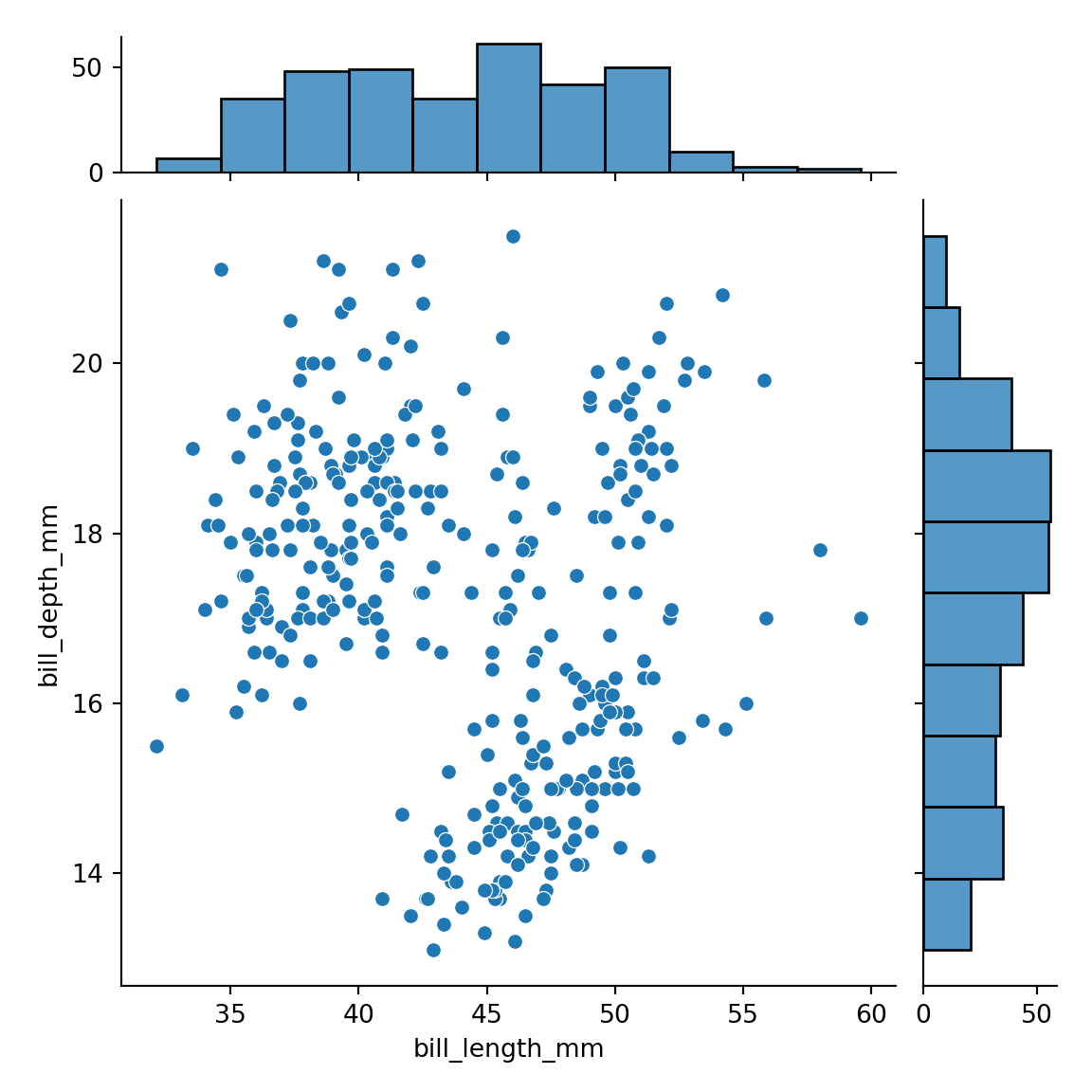Marginal plots size

By default, the scatter plot is 5 times bigger than the marginal plots. You can change the aspect ratio with ratio. Note that this argument only allows integers as input.

import seaborn as sns

# Scatter plot two times bigger than the histograms
sns.jointplot(data = df,
x = "bill_length_mm",
y = "bill_depth_mm",
ratio = 2)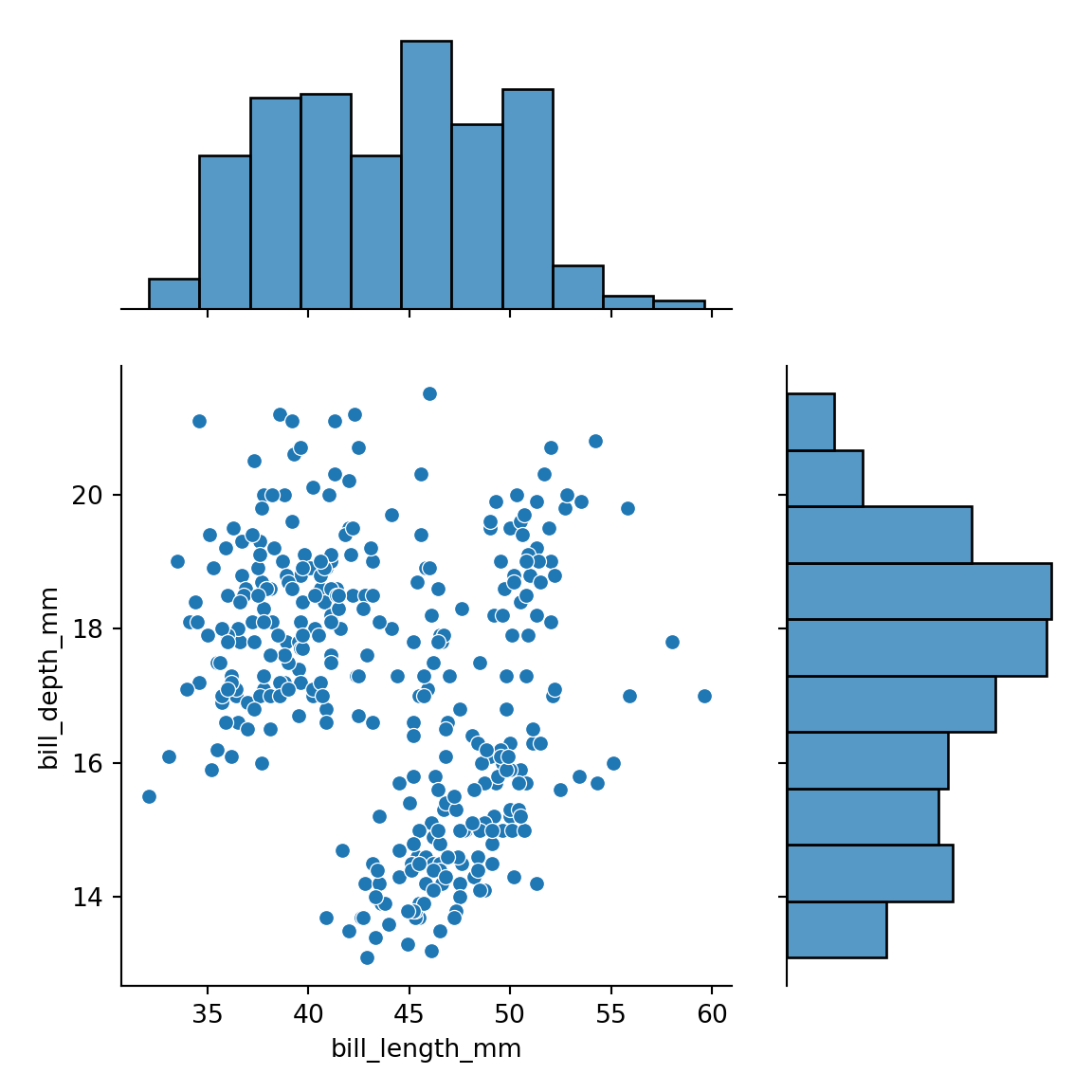### Color customization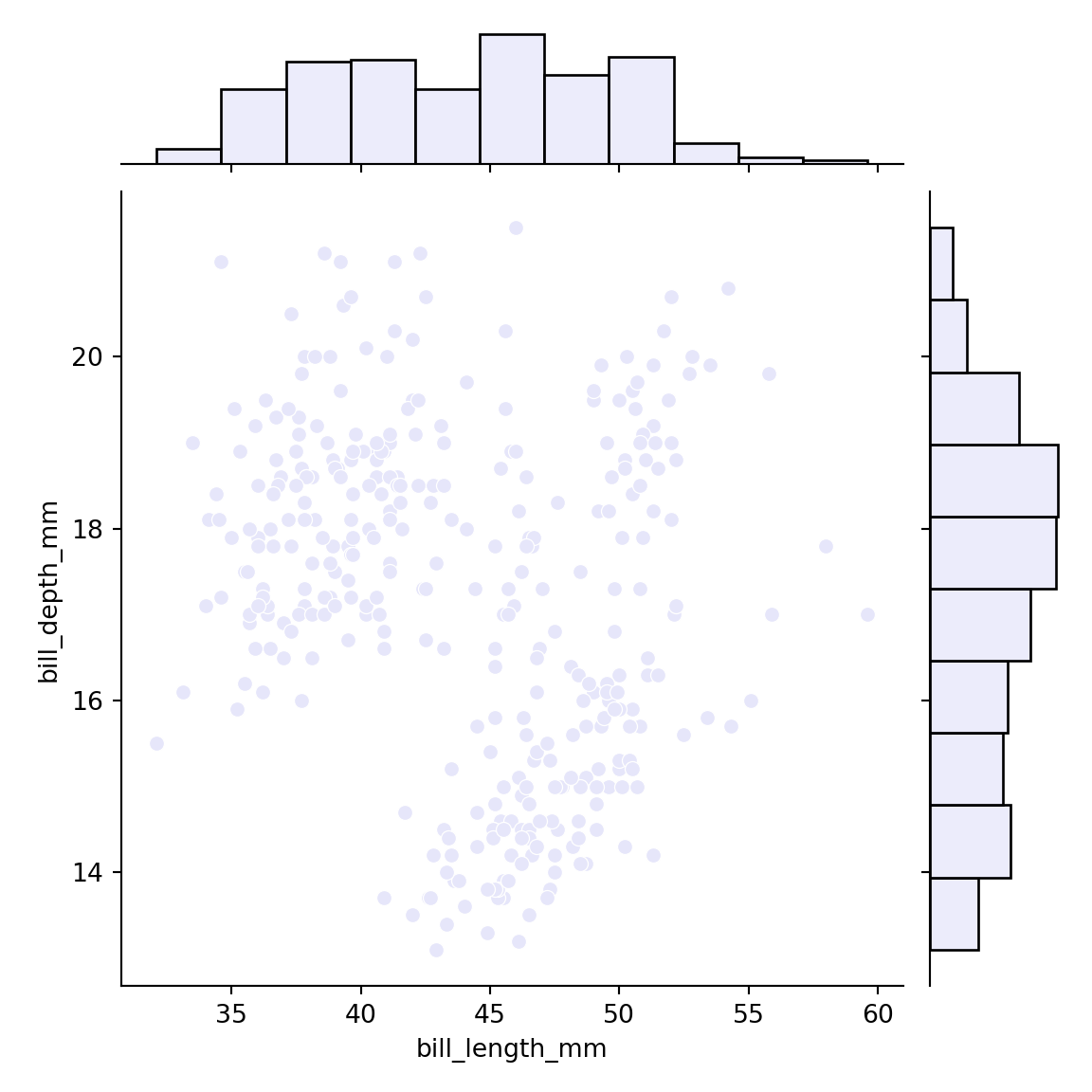Finally, you can also customize the colors. If you only provide a color using the color argument both the histograms and the scatter plot will have the same color.

import seaborn as sns

sns.jointplot(data = df,
x = "bill_length_mm",
y = "bill_depth_mm",
color = "lavender")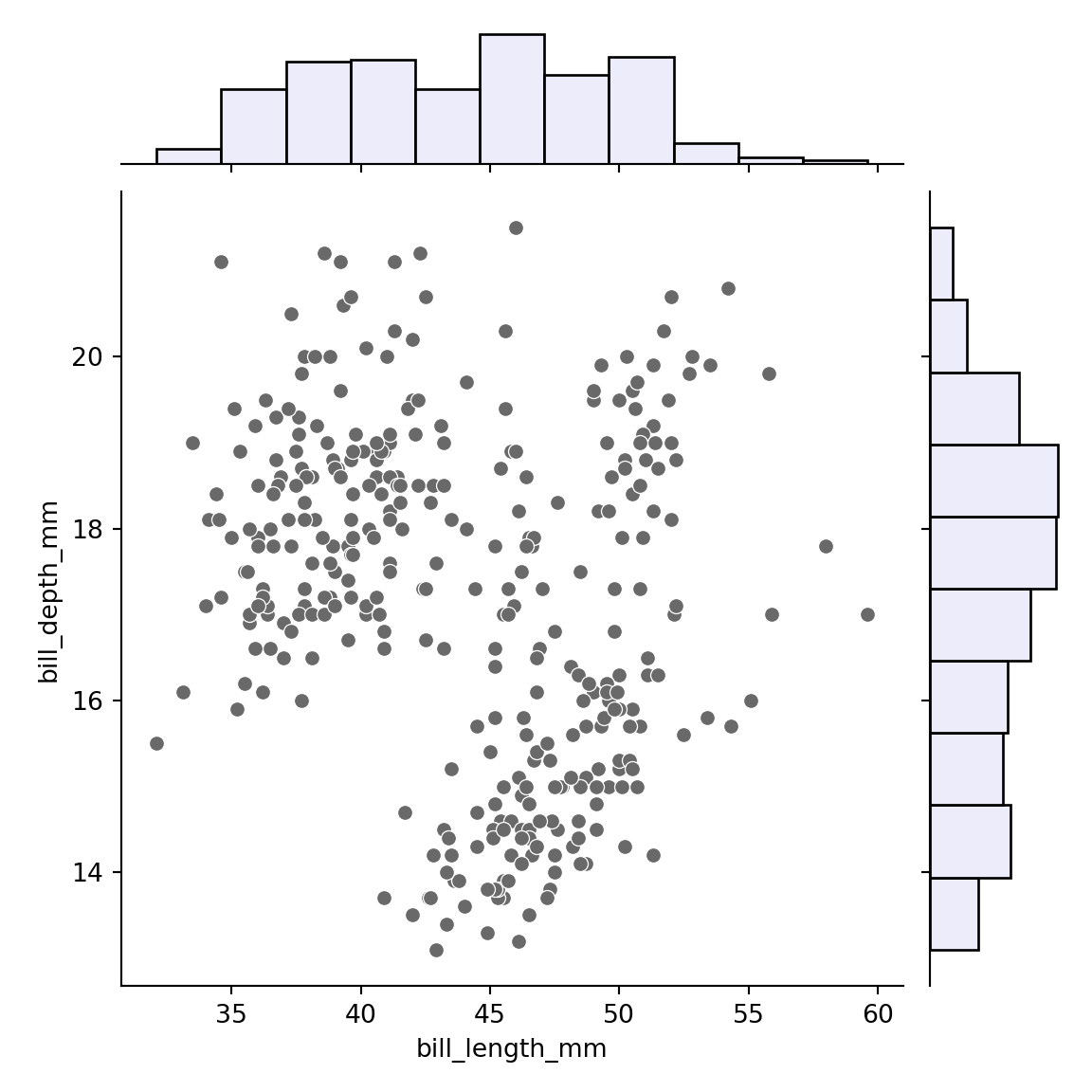However, if you prefer a different color for the histograms you can pass a dictionary to the marginal_kws argument and select the desired color. Note that this argument can also be used to customize other arguments related to the histograms.

import seaborn as sns

marginal_kws = dict(color = "lavender"))